Open in App
Not now

# Class 10 RD Sharma Solutions – Chapter 2 Polynomials – Exercise 2.3

• Last Updated : 20 May, 2021

### Question 1. Apply division algorithm to find the quotient q(x) and remainder r(x) on dividing f(x) by g(x) in each of the following:

(i) f(x) = x3 – 6x2 + 11x – 6, g(x) = x2 + x + 1

(ii) f(x) = 10x4 + 17x3 – 62x2 + 30x – 105, g(x) = 2x2 + 7x + 1

(iii) f(x) = 4x3 + 8x2 + 8x + 7, g(x) = 2x2 – x + 1

(iv) f(x) = 15x3 – 20x2 + 13x – 12, g(x) = x2 – 2x + 2

Solution:

(i) Here we have to divide f(x) = x3 – 6x2 + 11x – 6 by g(x) = x2 + x + 1

So, to get quotient q(x) and remainder r(x), we use division algorithm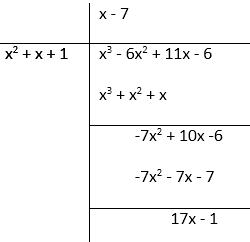Therefore,

Remainder r(x) = 17x – 1

Quotient q(x) = x – 7

(ii) Here we have to divide f(x) = 10x4 + 17x3 – 62x2 + 30x – 105 by g(x) = 2x2 + 7x + 1

So, to get quotient q(x) and remainder r(x), we use division algorithm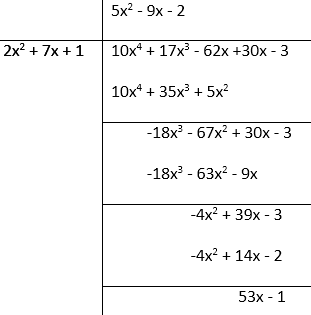Therefore,

Remainder r(x) = 53x – 1

Quotient q(x) = 5x2 – 9x – 2

(iii) Here we have to divide f(x) = 4x3 + 8x2 + 8x + 7 by g(x) =2x2 – x + 1

So, to get quotient q(x) and remainder r(x), we use division algorithm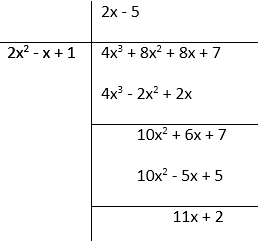Therefore,

Remainder r(x) = 11x + 2

Quotient q(x) = 2x – 5

(iv) f(x) = 15x3 – 20x2 + 13x – 12, g(x) = x2 – 2x + 2

Here we have to divide f(x) =  15x3 – 20x2 + 13x – 12 by g(x) = x2 – 2x + 2

So, to get quotient q(x) and remainder r(x), we use division algorithm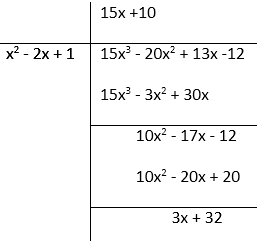Therefore,

Remainder r(x) = 3x + 32

Quotient q(x) = 15x + 10

### Question 2. Check whether the first polynomial is a factor of the second polynomial by applying the division algorithm:

(i) g(t) = t2 – 3; f(t) = 2t4 + 3t3 – 2t2 – 9t – 12

(ii) g(x) = x2 – 3x + 1; f(x) = x5 – 4x3 + x2 + 3x + 1

(iii) g(x) = 2x2 – x + 3; f(x) = 6x5 − x4 + 4x3 – 5x2 – x – 15

Solution:

(i) Here, we have to check whether g(t) = t2 – 3 is a factor of f(t) = 2t4 + 3t3 – 2t2 – 9t – 12

So, by using division algorithm, we get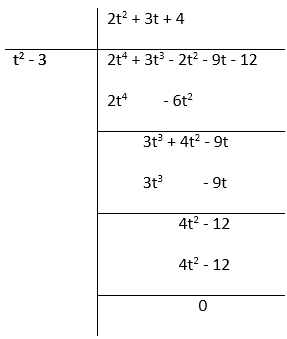As the remainder left is 0.

Therefore,

g(t) = t2 – 3 is a factor of f(t) = 2t4 + 3t3 – 2t2 – 9t – 12

(ii) Here, we have to check whether g(x) = x2 – 3x + 1 is a factor off(x) = x5 – 4x3 + x2 + 3x + 1

So, by using division algorithm, we get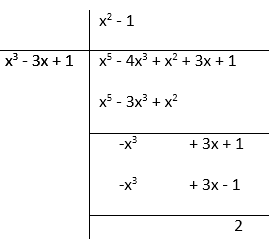As the remainder left is 2.

Therefore,

g(x) = x2 – 3x + 1 is not a factor of  f(x) = x5 – 4x3 + x2 + 3x + 1

(iii) Here, we have to check whether g(x) = 2x2 – x + 3 is a factor of f(x) = 6x5 − x4 + 4x3 – 5x2 – x – 15

So, by using division algorithm, we get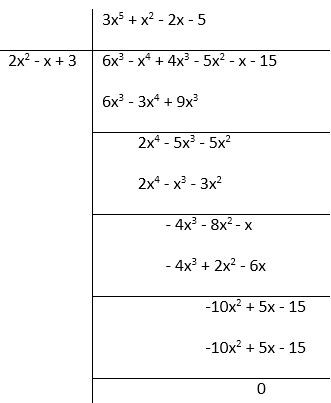As the remainder left is 0.

Therefore,

g(x) = 2x2 – x + 3 is a factor of f(x) = 6x5 − x4 + 4x3 – 5x2 – x – 15

### Question 3. Obtain all zeroes of the polynomial f(x) = f(x) = 2x4 + x3 – 14x2 – 19x – 6, if two of its zeroes are -2 and -1.

Solution:

Given: f(x) = 2x4 + x3 – 14x2 – 19x – 6

Here we have given the two zeroes of the polynomial that are -2 and -1,

Hence, its factors will be  (x + 2) and (x + 1)

Further,

(x + 2)(x + 1) = x2 + x + 2x + 2 = x2 + 3x + 2

So, by using division algorithm, we get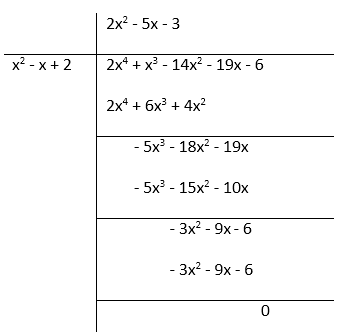f(x) = 2x4 + x3 – 14x2 – 19x – 6 = (2x2 – 5x – 3)(x2 + 3x + 2)

= (2x + 1)(x – 3)(x + 2)(x + 1)

Hence, the factors of f(x) = 2x4 + x3 – 14x2 – 19x – 6 are (2x + 1), (x – 3), (x + 2), (x + 1)

Therefore, the zeroes of the polynomial are -1/2, 3, -2, -1

### Question 4. Obtain all zeroes of f(x) = x3 + 13x2 + 32x + 20, if one of its zeroes is -2.

Solution:

We have been given the zero of the polynomial f(x) = x3 + 13x2 + 32x + 20 is -2.

Hence, its factor is (x + 2).

So, by using division algorithm, we get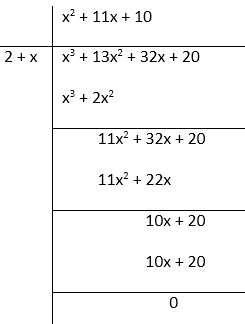Thus,

f(x) = x3 + 13x2 + 32x + 20

= (x2 + 11x + 10)(x + 2)

= (x2 + 10x + x + 10)(x + 2)

= (x + 10)(x + 1)(x + 2)

Hence, the factors of f(x) = x3 + 13x2 + 32x + 20 are (x + 10), (x + 1), (x + 2)

Thus, the zeroes of the polynomial are -1, -10, -2.

### Question 5. Obtain all zeroes of the polynomial f(x) = x4 – 3x3 – x2 + 9x – 6, if the two of its zeroes are -√3 and √3.

Solution:

Here, we are given two zeros of the polynomial f(x) = x4 – 3x3 – x2 + 9x – 6 that are -√3 and √3.

Thus, the factors are (x + √3​)(x − √3​) ⇒ x2 – 3.

So, by using division algorithm, we get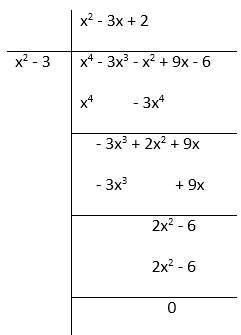Hence,

f(x) = x4 – 3x2 – x2 + 9x – 6 = (x2 – 3)(x2 – 3x + 2)

(x + √3)(x – √3)(x2 – 2x – 2 + 2)

= (x + √3)(x – √3)(x – 1)(x – 2)

Thus, the factors of f(x) = x4 – 3x3 – x2 + 9x – 6 are (x + √3)(x – √3)(x – 1)(x – 2).

Therefore, the zeroes of the polynomial are -√3, √3, 1, 2.

### Question 6. Obtain all zeroes of the polynomial f(x) = 2x4 – 2x3 – 7x2 + x – 1, if the two of its zeroes are -√3/2 and √3/2.

Solution:

Here, we are given two zeros of the polynomial f(x) = 2x4 – 2x3 – 7x2 + x – 1 that are -√3/2 and √3/2.

Thus, the factors are⇒ x2 – 3/2.

So, by using division algorithm, we get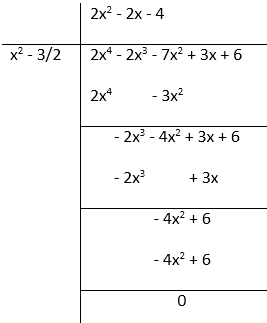Hence,Factors of f(x) = 2x4 – 2x3 – 7x2 + x – 1 are.

Thus, the zeroes of the polynomial are -1, 2, -√3/2 and √3/2.

### Question 7. Find all the zeroes of the polynomial x4 + x3 – 34x2 – 4x + 120, if the two of its zeroes are 2 and – 2.

Solution:

Here, we are given two zeros of the polynomial x4 + x3 – 34x2 – 4x + 120 that are 2 and -2.

Thus, the factors are (x + 2)(x – 2)⇒ x2 – 4.

So, by using division algorithm, we get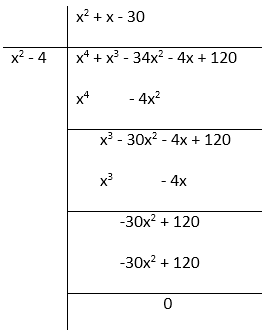Hence,

x4 + x3 – 34x2 – 4x + 120 = (x2 – 4)(x2 + x – 30)

= (x – 2)(x + 2)(x2 + 6x – 5x – 30)

=  (x – 2)(x + 2)(x + 6)(x – 5)

So, the factors of x4 + x3 – 34x2 – 4x + 120 are (x – 2), (x + 2), (x + 6), (x – 5)

Thus, the zeroes of the polynomial = x = 2, – 2, – 6, 5

### Question 8. Find all the zeroes of the polynomial 2x4 + 7x3 – 19x2 – 14x + 30, if the two of its zeroes are √2 and -√2.

Solution:

Here, we are given two zeros of the polynomial 2x4 + 7x3 – 19x2 – 14x + 302 that are √2 and -√2.

Thus, the factors are (x + √2)(x – √2) ⇒ x2 – 2.

So, by using division algorithm, we get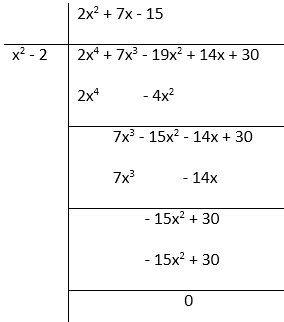Hence,

2x4 + 7x3 – 19x2 – 14x + 30 = (x2 – 2)(2x2 + 7x – 15)

= (2x2 + 10x – 3x – 15)(x + √2)(x – √2)

= (2x – 3)(x + 5)(x + √2)(x – √2)

So, the factors of 2x4 + 7x3 – 19x2 – 14x + 30 are (2x – 3), (x + 5), (x + √2), (x – √2)

Thus, the zeroes of the polynomial is √2, -√2, -5, 3/2.

### Question 9. Find all the zeroes of the polynomial f(x) = 2x3 + x2 – 6x – 3, if two of its zeroes are -√3 and √3.

Solution:

Here, we are given two zeros of the polynomial f(x) = 2x3 + x2 – 6x – 3 that are -√3 and √3.

Thus, the factors are (x + √3)(x – √3) ⇒ x2 – 3.

So, by using division algorithm, we get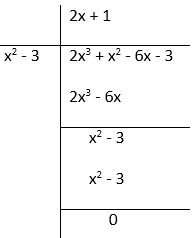Hence,

f(x) = 2x3 + x2 – 6x – 3

= (x2 – 3)(2x + 1)

= (x + √3)(x – √3)(2x + 1)

Factors of f(x) = 2x3 + x2 – 6x – 3 are (x + √3), (x – √3), 2x + 1

Thus, the zeroes for the given polynomial are √3, -√3, -1/2

### Question 10. Find all the zeroes of the polynomial f(x) = x3 + 3x2 – 2x – 6, if the two of its zeroes are √2 and -√2.

Solution:

Here, we are given two zeros of the polynomial f(x) = x3 + 3x2 – 2x – 6 that are √2 and -√2.

Thus, the factors are (x + √2)(x – √2)⇒ x2 – 2.

So, by using division algorithm, we get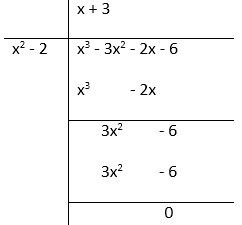Hence,

f(x) = x3 + 3x2 – 2x – 6

= (x2 – 2)(x + 3)

= (x + √2)(x – √2)(x + 3)

Factors of f(x) = x3 + 3x2 – 2x – 6 are (x + √2), (x – √2), (x + 3)

Thus, the zeroes of the given polynomial is -√2, √2, and – 3.

### Question 11. What must be added to the polynomial f(x) = x4 + 2x3 – 2x2 + x − 1 so that the resulting polynomial is exactly divisible by g(x) = x2 + 2x − 3.

Solution:

Here we have to add to the polynomial f(x) = x4 + 2x3 – 2x2 + x − 1 so that the

resulting polynomial is exactly divisible by g(x) = x2 + 2x − 3.

So, divide f(x) = x4 + 2x3 – 2x2 + x − 1 by g(x) = x2 + 2x − 3 to get the answer.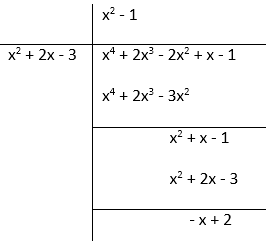As the remainder left is (x – 2) to get the resulting polynomial exactly divisible by

g(x) = x2 + 2x − 3 we must add (x – 2) to f(x) = x4 + 2x3 – 2x2 + x − 1.

### Question 12. What must be subtracted from the polynomial f(x) = x4 + 2x3 – 13x2 –12x + 21 so that the resulting polynomial is exactly divisible by g(x) = x2 – 4x + 3.

Solution:

Here we have to subtract to the polynomial f(x) = x4 + 2x3 – 13x2 – 12x + 21

so that the resulting polynomial is exactly divisible by g(x) = x2 – 4x + 3.

So, divide f(x) = x4 + 2x3 – 13x2 – 12x + 21 by  g(x) = x2 – 4x + 3 to get the answer.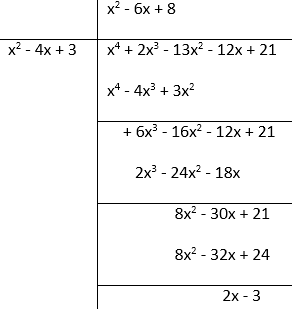As the remainder left is (2x – 3) to get the resulting polynomial exactly divisible by

g(x) = x2 – 4x + 3 we must add (2x – 3) to f(x) = x4 + 2x3 – 13x2 – 12x + 21.

### Question 13. Given that √2 is a zero of the cubic polynomial f(x) = 6x3 + √2x2– 10x – 4√2, find its other two zeroes.

Solution:

Here, we are given that √2 is the zero of the cubic polynomial

f(x) = 6x3 + √2x2– 10x – 4√2, thus, factor of the polynomial is (x – √2)

So, by using division algorithm, we get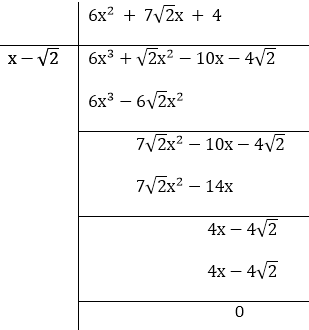Hence,

f(x) = 6x3 + √2x2 – 10x – 4√2

= (x – √2)(6x2 + 7√2x + 4)

= (x – √2)(6x2 + 4√2x + 3√2x + 4)

= (x – √2)(3x + 2√2)(2x + √2)

The factors of f(x) = 6x3 + √2x2– 10x – 4√2 are (x – √2), (3x + 2√2), (2x + √2)

Therefore, the zeros of the polynomial are -2√2/3, -√2/2, √2

### Question 14. Given that x – √5 is a factor of the cubic polynomial x3 – 3√5x2 + 13x – 3√5, find all the zeroes of the polynomial.

Solution:

Here, we have x – √5 as factor of the cubic polynomial x3 – 3√5x2 + 13x – 3√5

To find all the zeros of the polynomial, we have to divide the polynomial x3 – 3√5x2 + 13x – 3√5 by the factor x – √5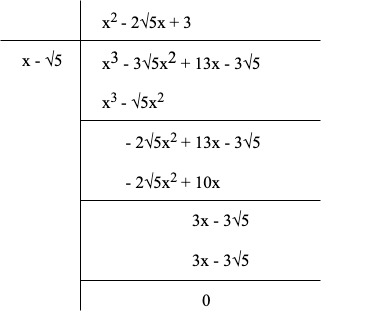Hence,

x3 – 3√5x2 + 13x – 3√5

= (x – √5)(x2 – 2√5 + 3)

= (x – √5)(x – (√5 + √2))(x – (√5 – √2))

So, the factors of the cubic polynomial x3 – 3√5x2 + 13x – 3√5 are (x – √5), (x – (√5 + √2)), (x – (√5 – √2))

Therefore, the zero of the polynomial are √5, (√5 – √2), (√5 + √2)

My Personal Notes arrow_drop_up